//没登陆

 开荒之星勋章申请：组图打开中，请稍候......符文发放器 + 110 活动奖励

[armory]http://hero.d.163.com/hero/cn/xielz-5495/381391[/armory]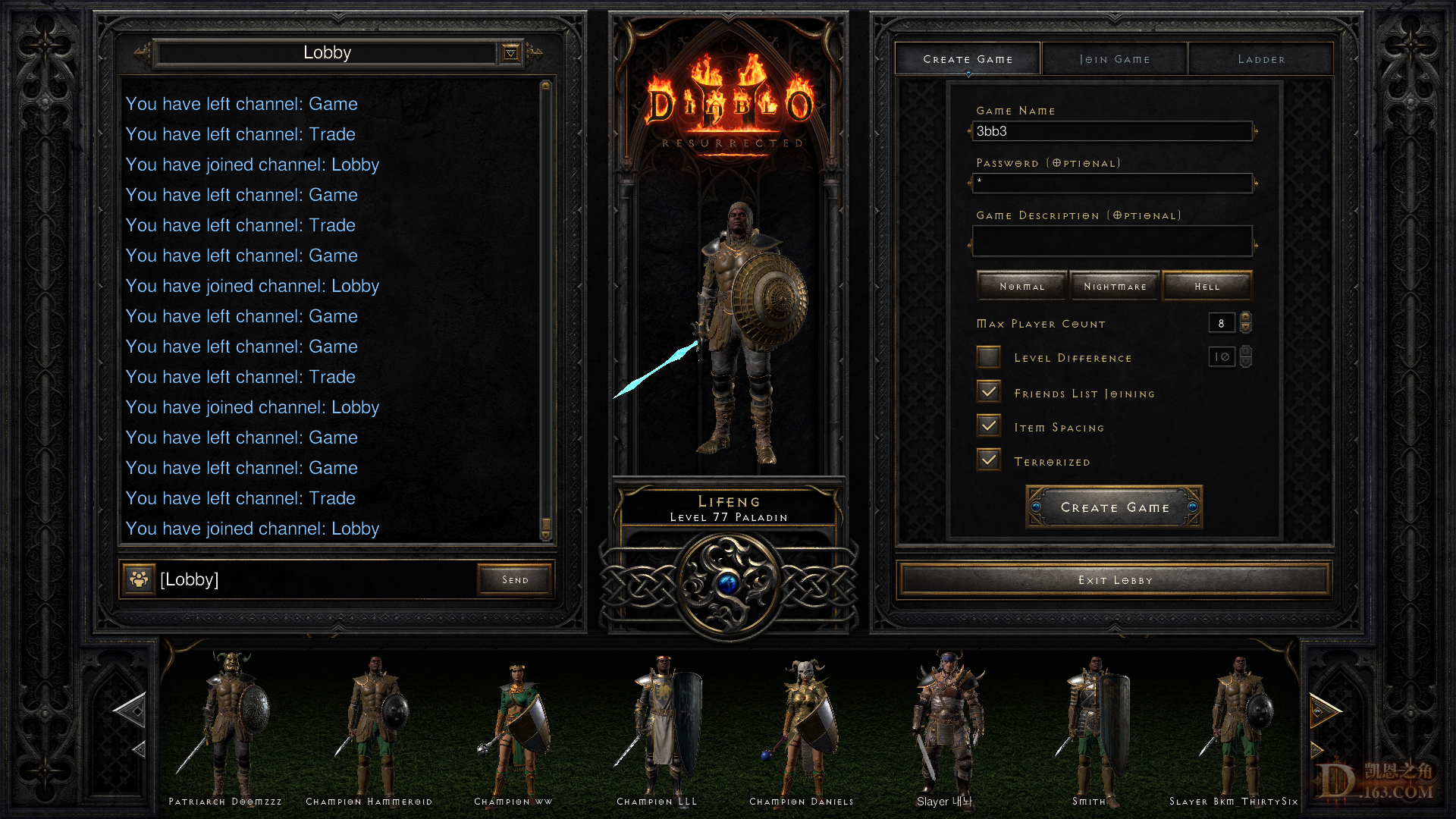完成开荒任务。。。。。。。。符文发放器 + 110 活动奖励

 参加活动：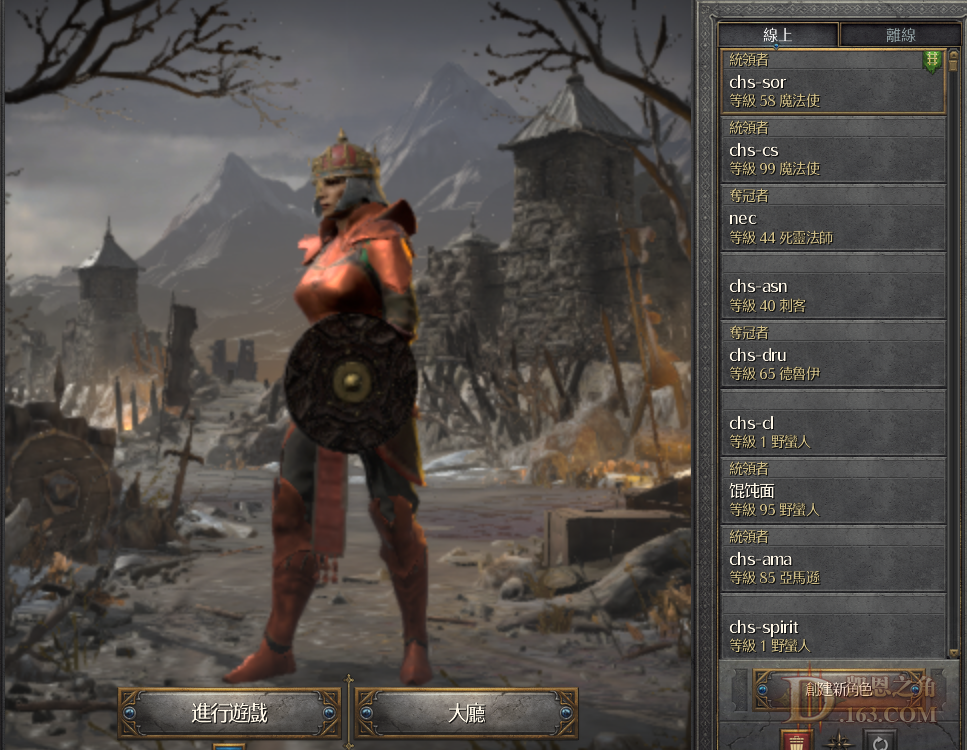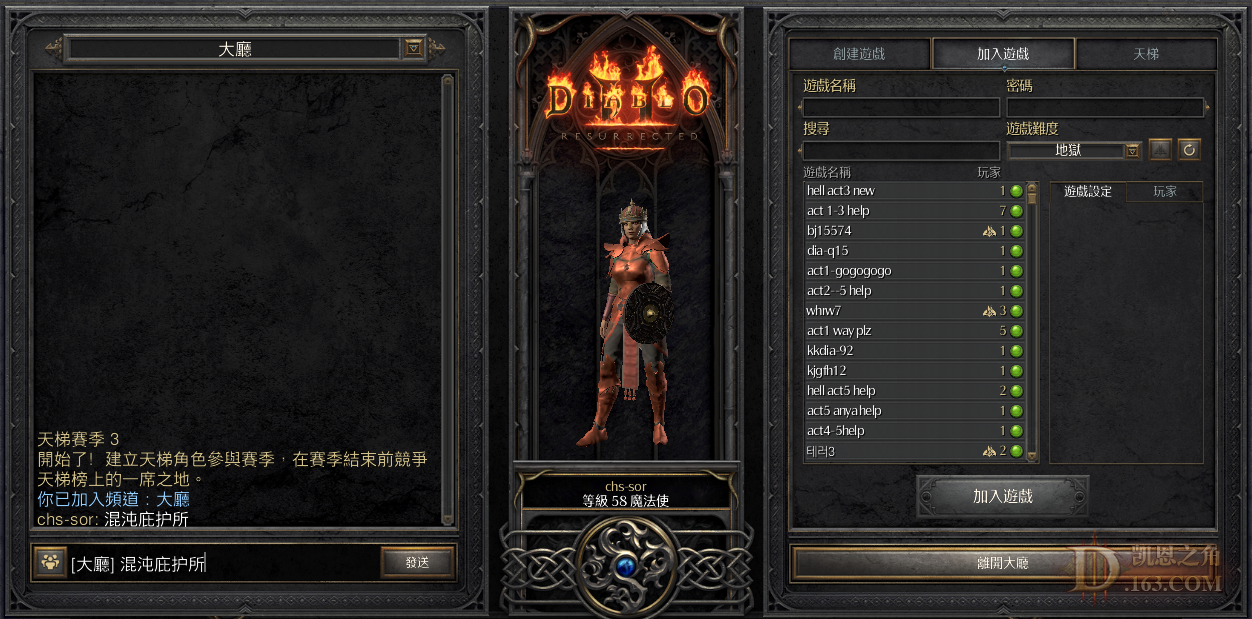符文发放器 + 10 活动奖励

tag: hetao#21196
 开荒之星勋章申请：组图打开中，请稍候......离开家 + 10 活动奖励

 本帖最后由 流火未泮 于 2023-2-19 04:15 编辑 开荒之星勋章申请：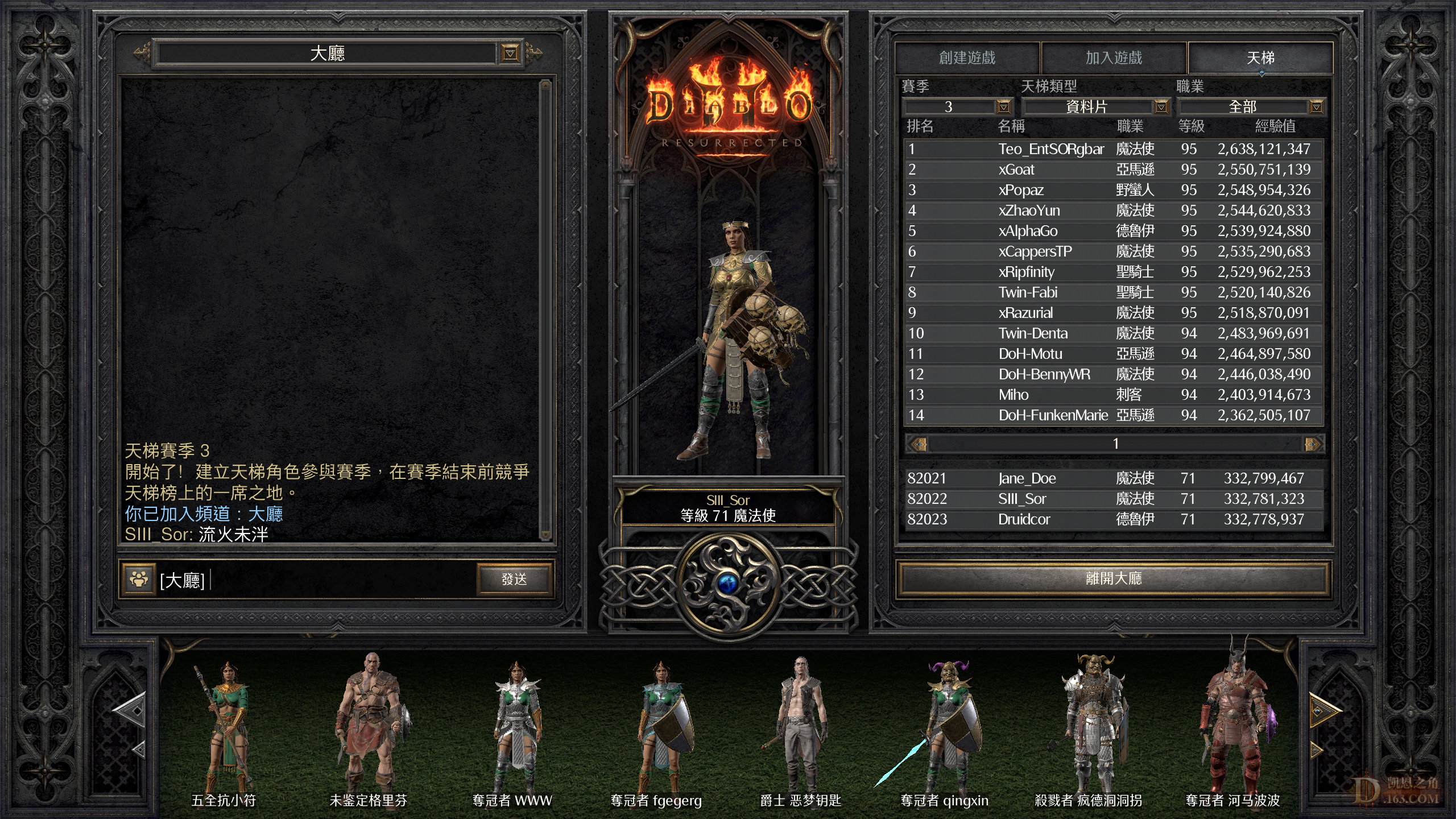符文发放器 + 110 活动奖励

 本帖最后由 多姿多彩 于 2023-2-19 04:25 编辑 开荒之星勋章申请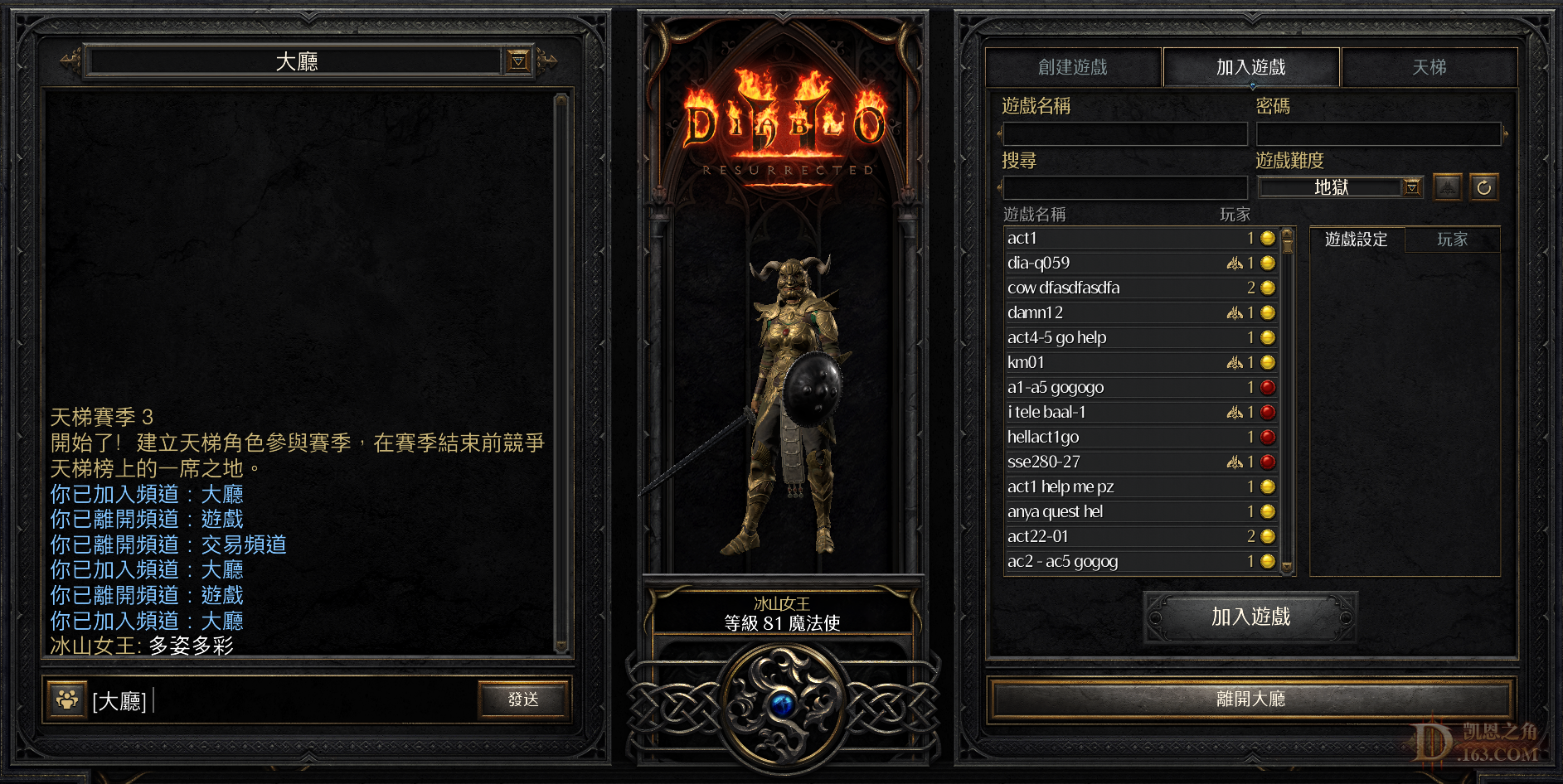符文发放器 + 110 活动奖励

 开荒之星勋章申请：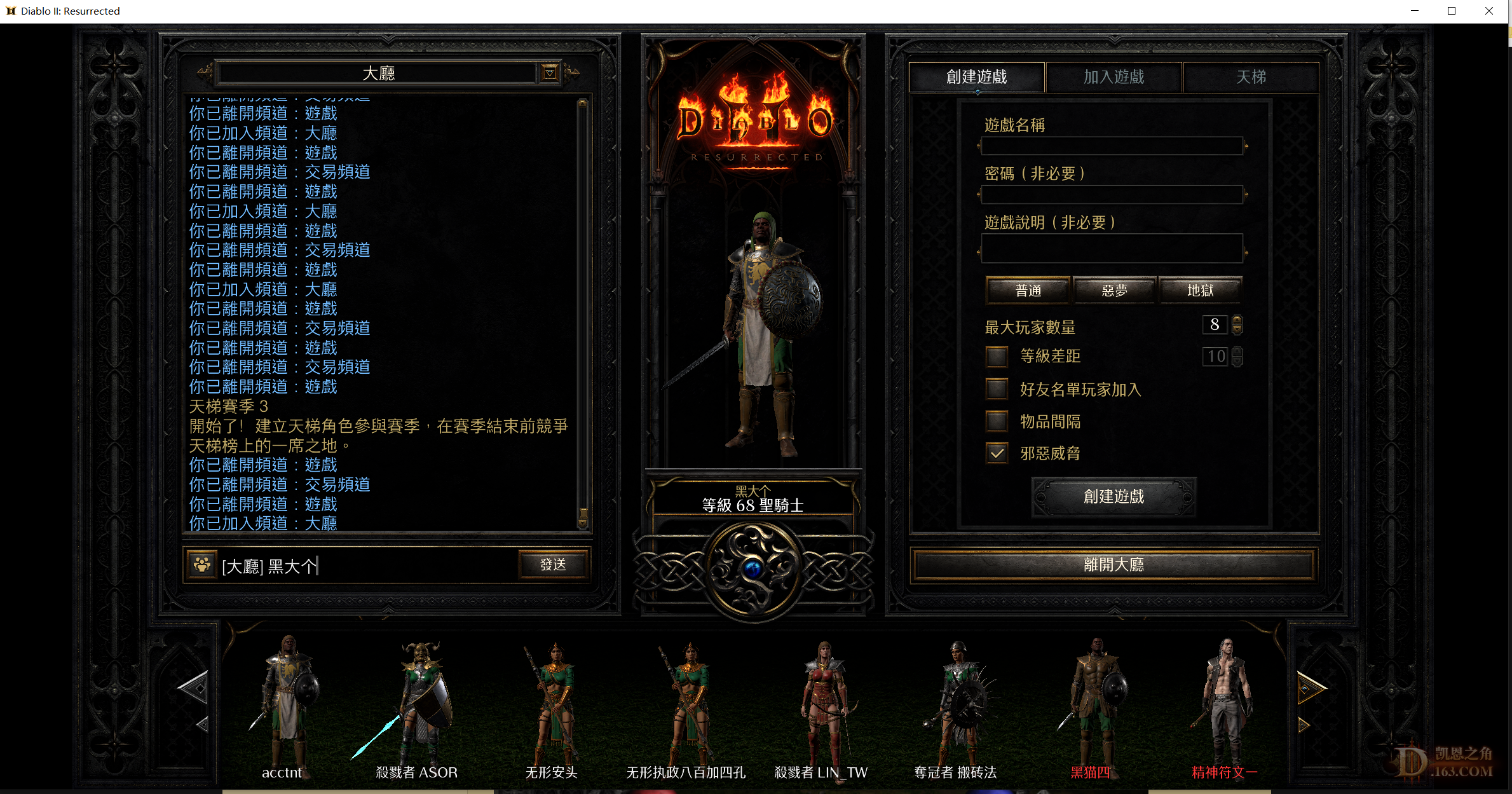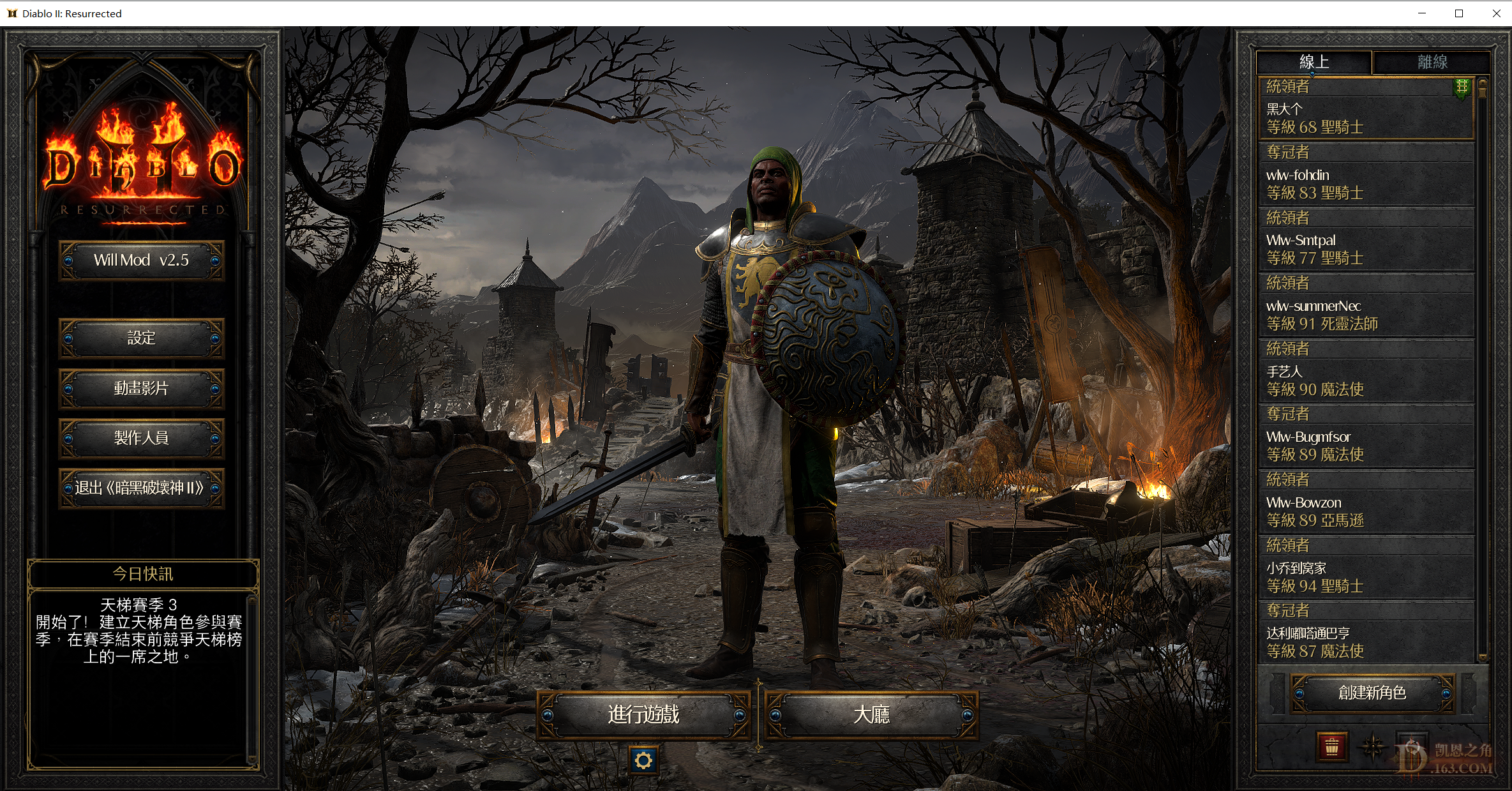符文发放器 + 110 活动奖励

wlwsor#3155
S0：24=2 25=7 26=2 27=2 28=1 29=3 30=1
31=2 32=1 33=1
S1\rune：32=1 31=2 30=1 29=2  28=1
27=2 25=3 24=4//15/15 mp+/200/9 wxtt/ 3/19/20 sc
S3\rune：24=2 28=2 29=2 31=1 //451pd sc
S4\rune:  26=1 31=1//glf=2 py=1 nj=1 15/15 eth td
 开荒之星勋章申请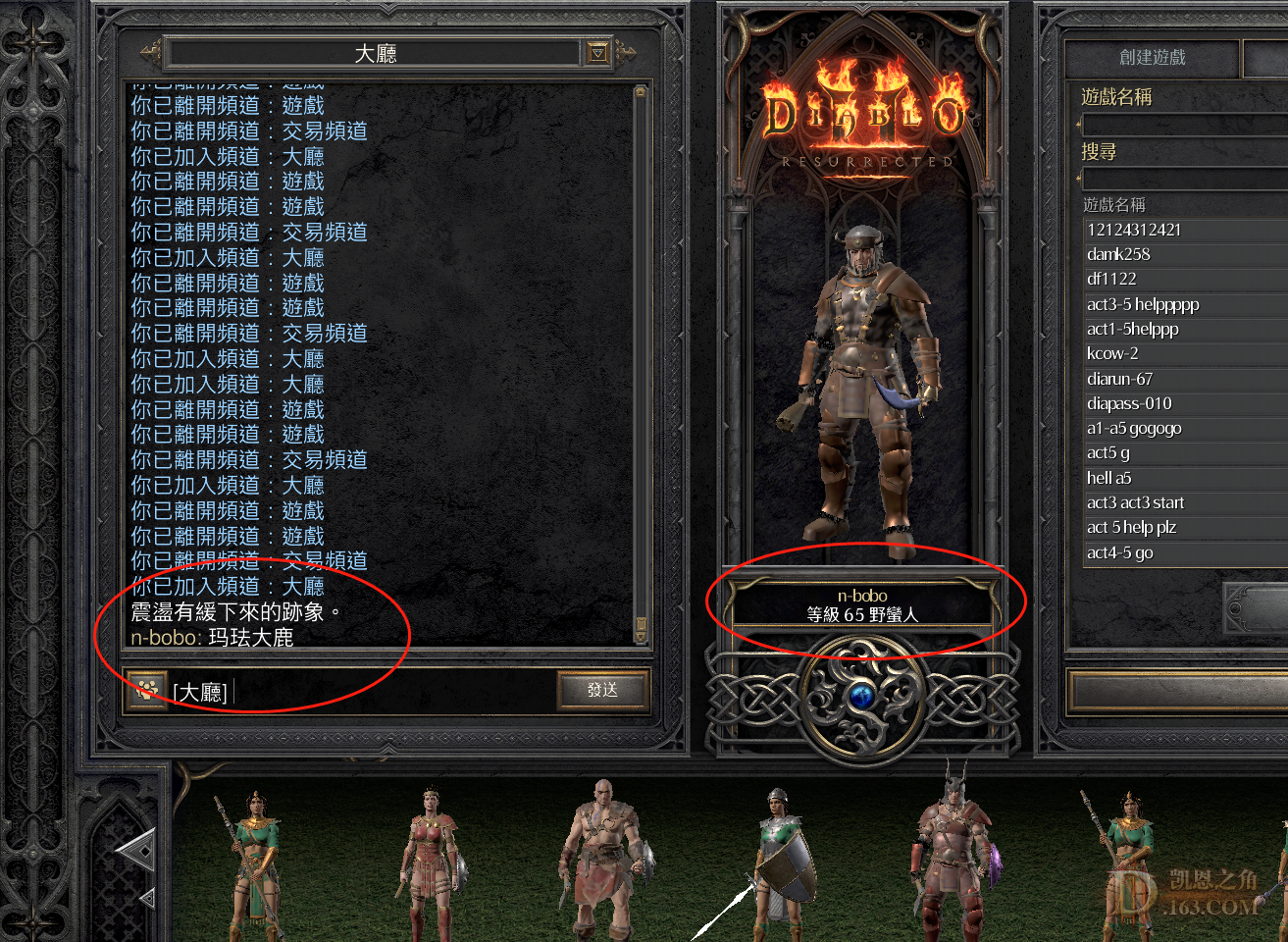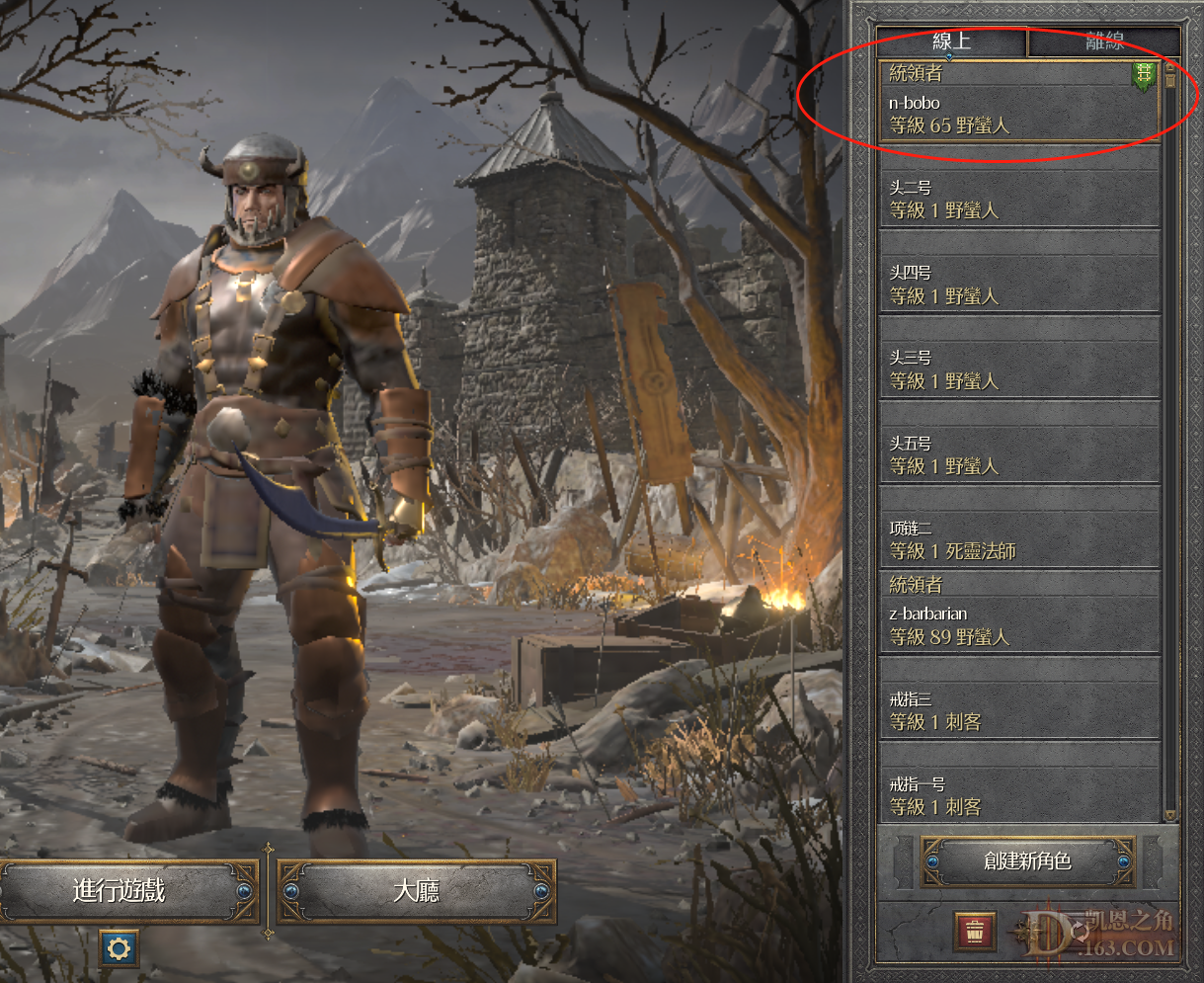离开家 + 10 活动奖励

niko#31828
 本帖最后由 dyqr 于 2023-2-19 07:12 编辑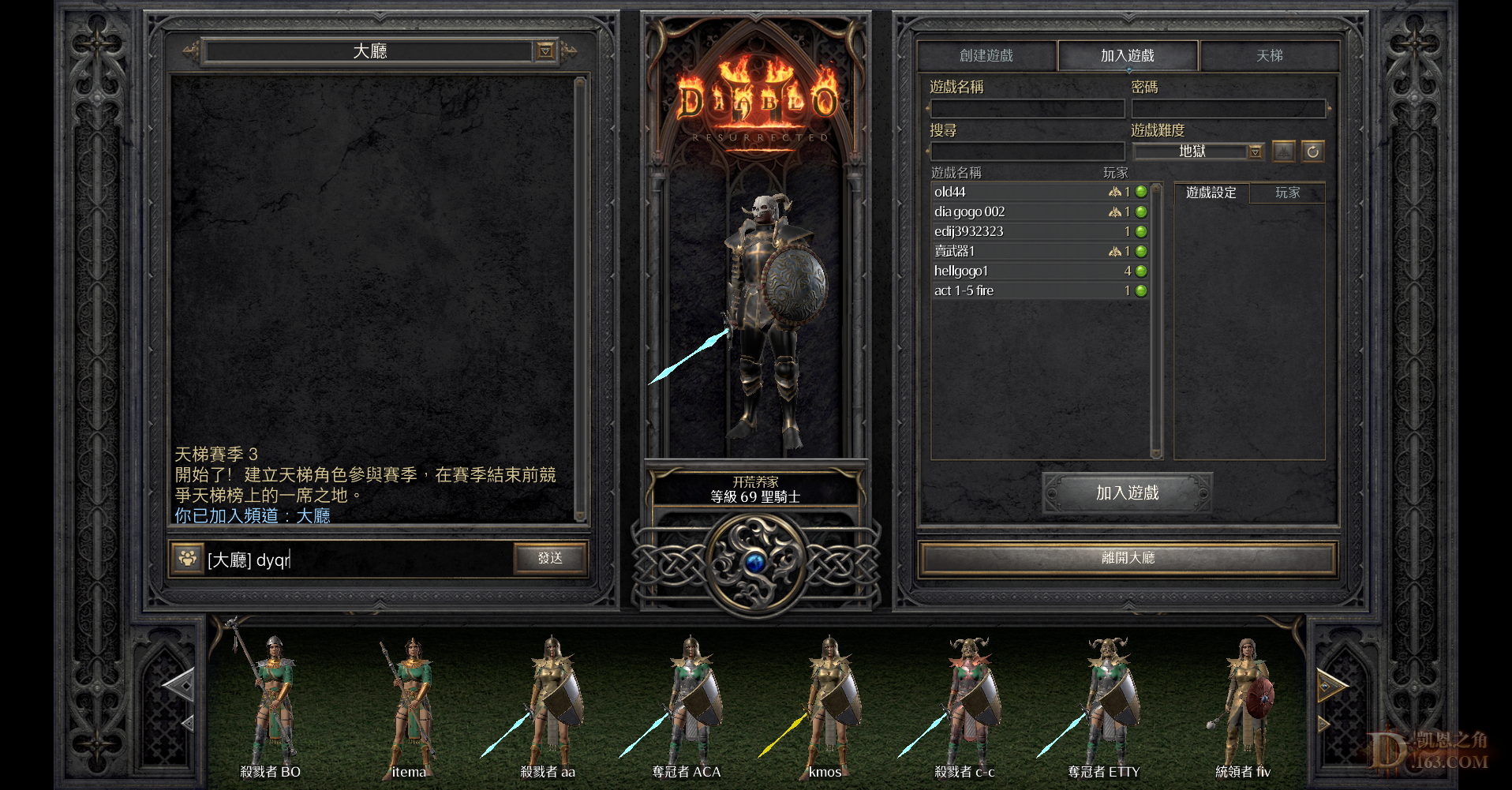离开家 + 10 活动奖励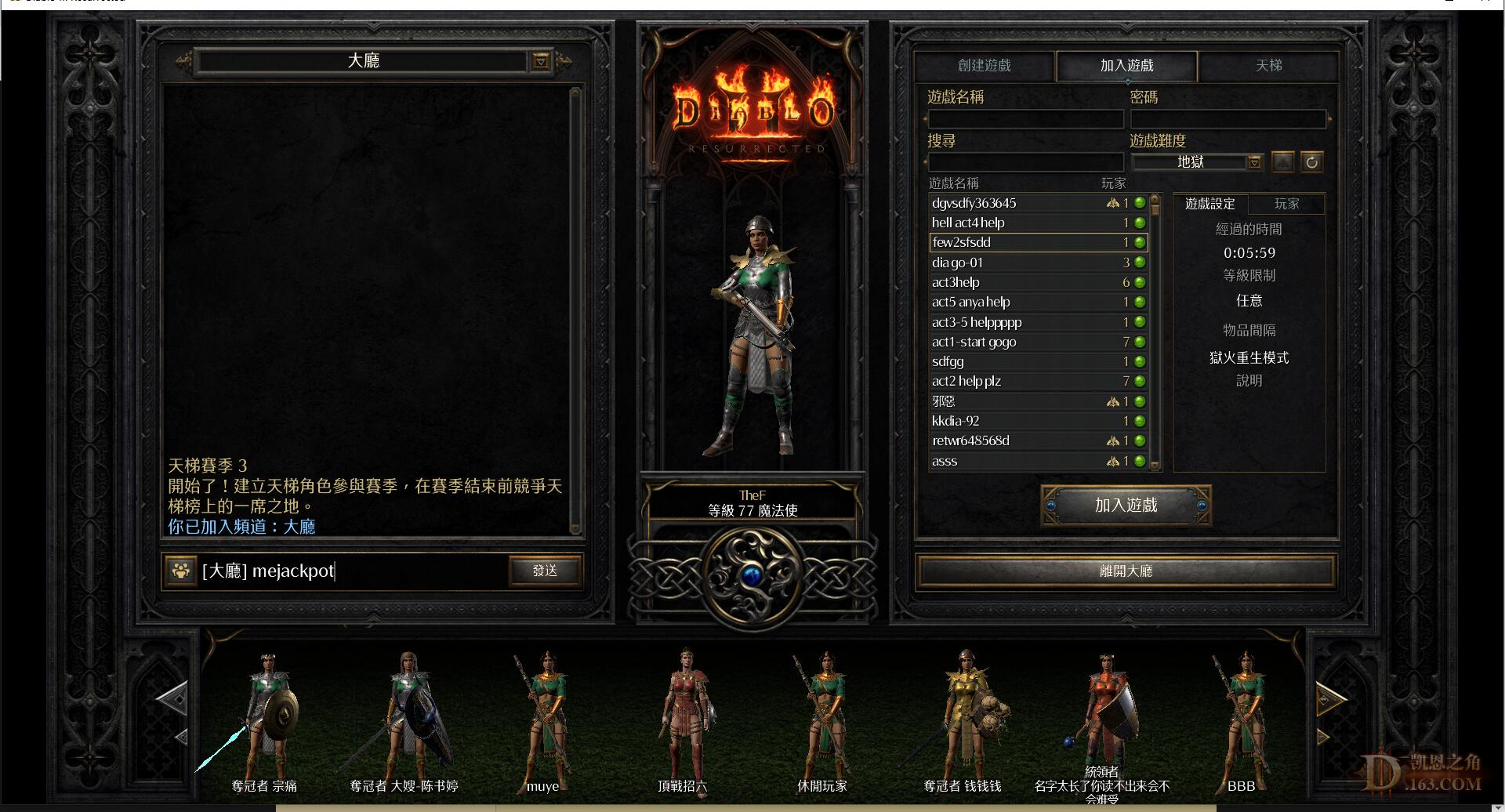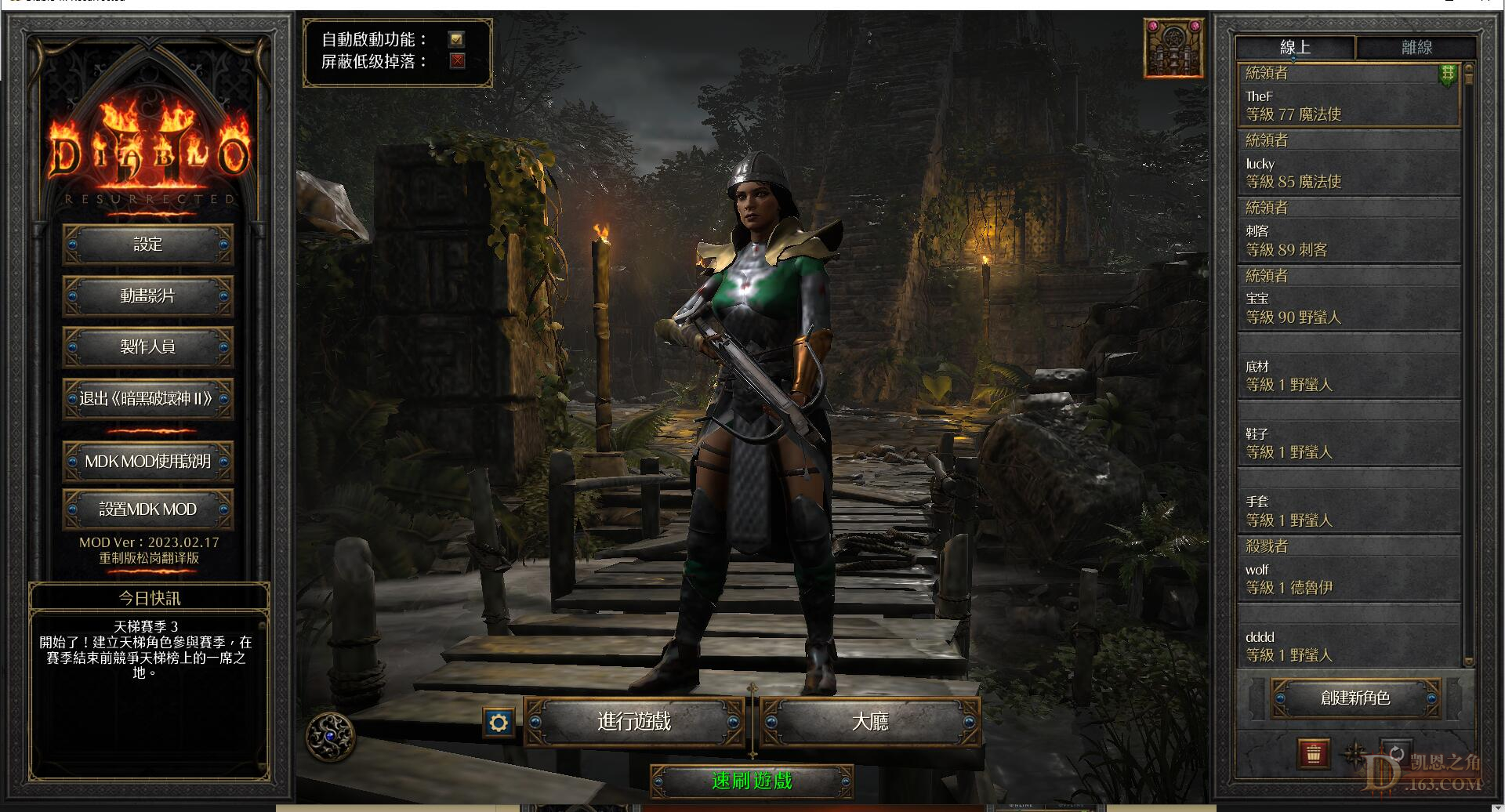离开家 + 10 活动奖励

mejackpot#31709
 开荒之星勋章申请：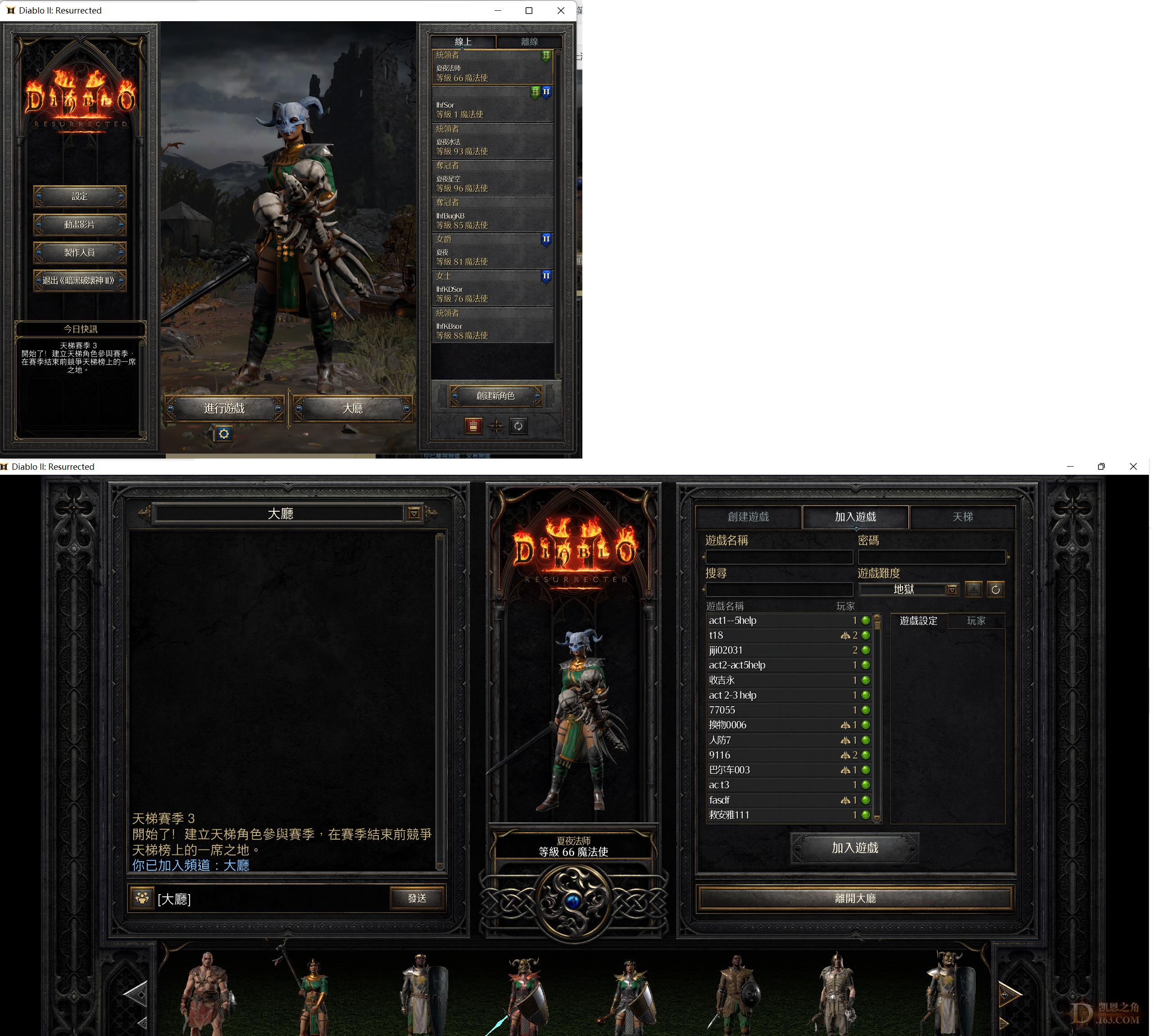离开家 + 10 活动奖励

https://locimg.com/
 开荒之星勋章申请组图打开中，请稍候......离开家 + 10 活动奖励开荒之星勋章申请离开家 + 10 活动奖励

 申请开荒之星勋章~~~~~~~~~~~~组图打开中，请稍候......离开家 + 10 活动奖励

 开荒之星勋章申请组图打开中，请稍候......离开家 + 10 活动奖励